## Google Sheets Query function: The Most Powerful Function in Google Sheets

Have you heard of the Google Sheets Query function?

It allows you to use powerful database code (a pseudo-SQL, Structured Query Language, the code used to communicate with databases) to manipulate your data in Google Sheets and it’s SUPER powerful.

It’s arguably the most powerful function in Google Sheets.

This video is lesson 14 of 30 from my free Google Sheets course: Advanced Formulas 30 Day Challenge

(The tutorial below uses a different dataset.)

1. If you want to follow along and have the same data and see all of the formula solutions, click this link to make your own copy of the Google Sheet:

Grab the data and solution file for this tutorial:

This is what our starting data looks like: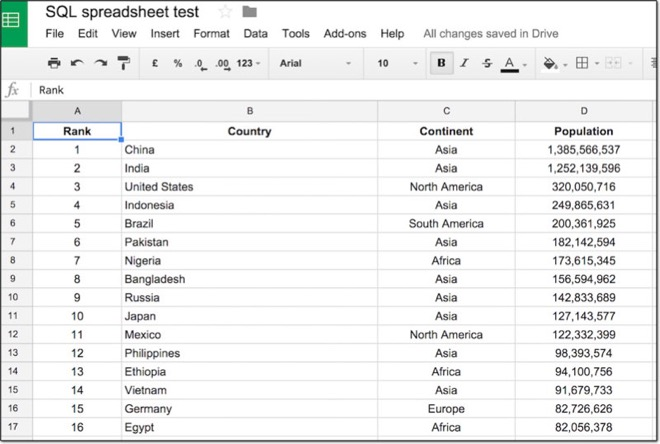2. Ensure you have the whole table selected (Pro-tip: click somewhere in your table and hit Ctrl + A (on PC) or Cmd + A (on Mac) to highlight the whole table):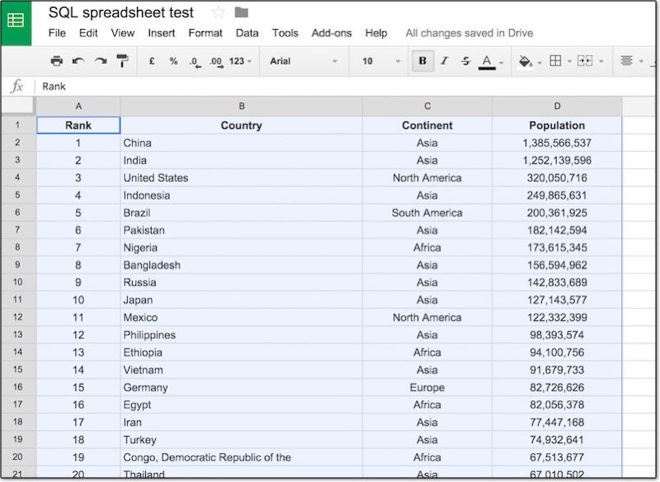3. Go to the menu: Data > Named ranges… and click this menu. A new pane will show in the right side of your spreadsheet as follows:4. In the first input box, enter the word “countries”, as shown in the image below. This names our table of data so we can refer to it easily.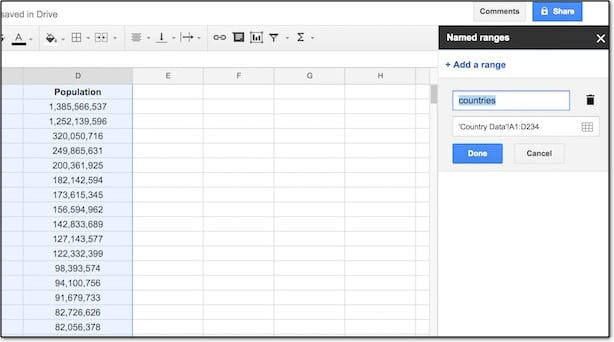5. In Google sheets we use the Google Sheets QUERY function and write our pseudo-SQL code inside this function. So, we’ll enter all of our SQL code inside a QUERY function in cell G1.

`=QUERY(countries,"our SQL code goes here between the quotes",1)`

Ok, now we’re set up, let’s start writing SQL code!

## SELECT all the data with the Google Sheets QUERY function

The SQL code `SELECT *` retrieves all of the columns from our data table.

6. To the right side of the table, type the following Google Sheets QUERY function into cell G1:

`=QUERY(countries,"SELECT *",1)`

7. The output from this query is our full table again, because `SELECT *` retrieves all of the columns from the countries table: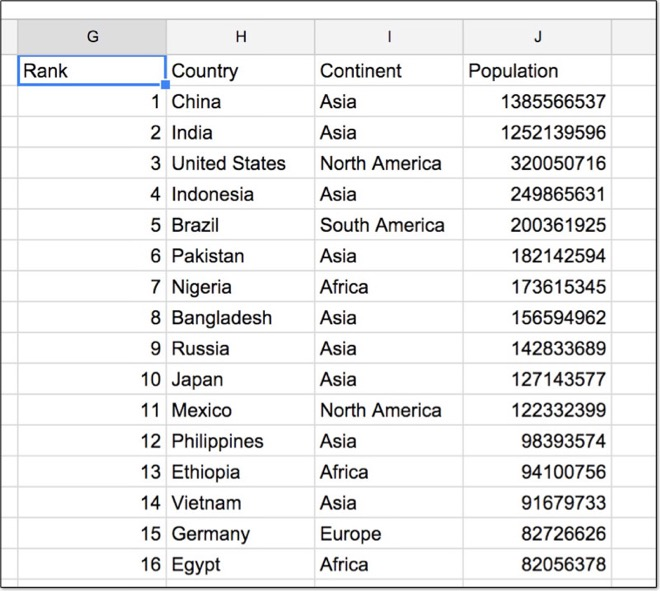Wow, there you go! You’ve written your first piece of SQL code! Pat yourself on the back.

The equivalent real world SQL code would be:

```SELECT * FROM countries```

Spot the difference: We don’t include a `FROM` clause with the Google Sheets QUERY function. This is different to SQL in the real world, where we must specify a `FROM` clause to select our table.

## SELECT specific columns only

8. What if we don’t want to select every column, but only certain ones? Modify your Google Sheets QUERY function to read:

`=QUERY(countries,"SELECT B, D",1)`

9. This time we’ve selected only columns B and D, so our output will look like this: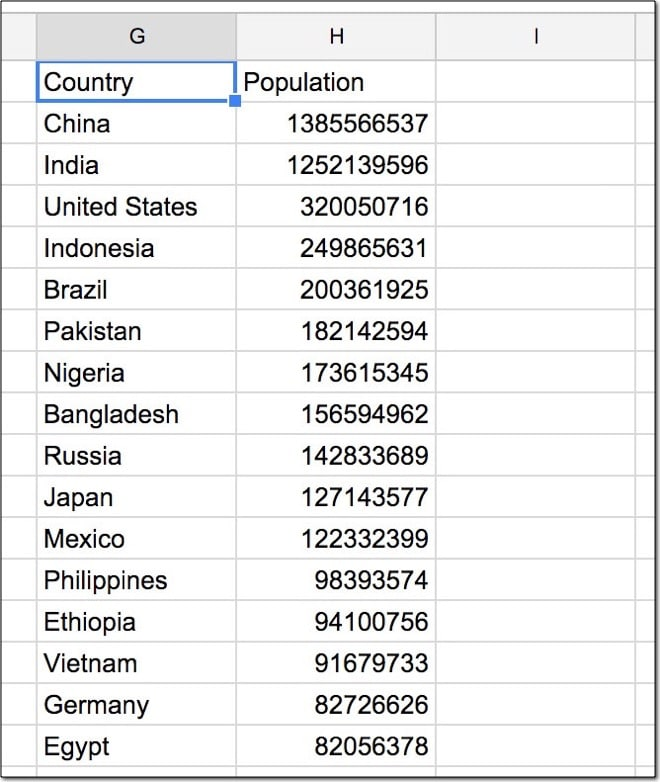Equivalent real world SQL code:

```SELECT country, population FROM countries```

Grab the data and solution file for this tutorial:

## WHERE clause

The WHERE clause specifies a condition that must be satisfied. It filters our data. It comes after the SELECT clause.

10. Modify your Google Sheets QUERY function in cell G2 to select only countries that have a population greater than 100 million:

`=QUERY(countries,"SELECT B, D WHERE D > 100000000",1)`

11. Our output table is: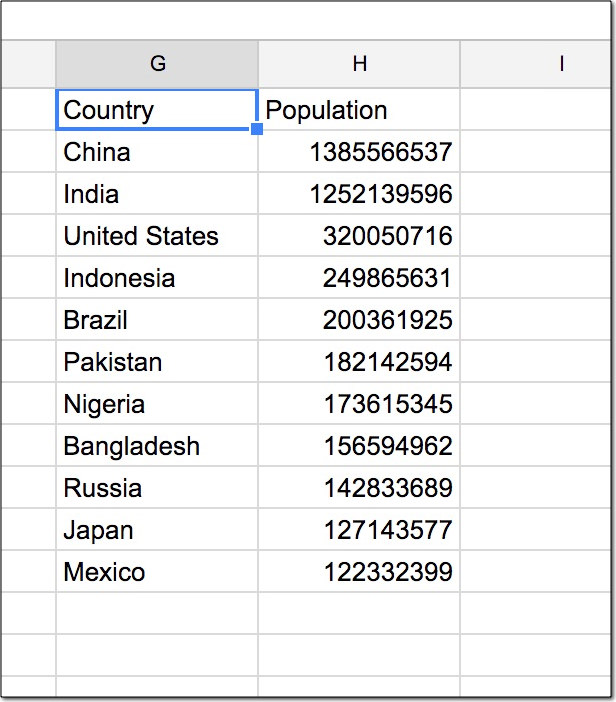Equivalent real world SQL code:

```SELECT country, population FROM countries WHERE population > 100000000```

12. Let’s see another WHERE clause example, this time selecting only European countries. Modify your formula to:

`=QUERY(countries,"SELECT B, C, D WHERE C = 'Europe' ",1)`

13. Now the output table is: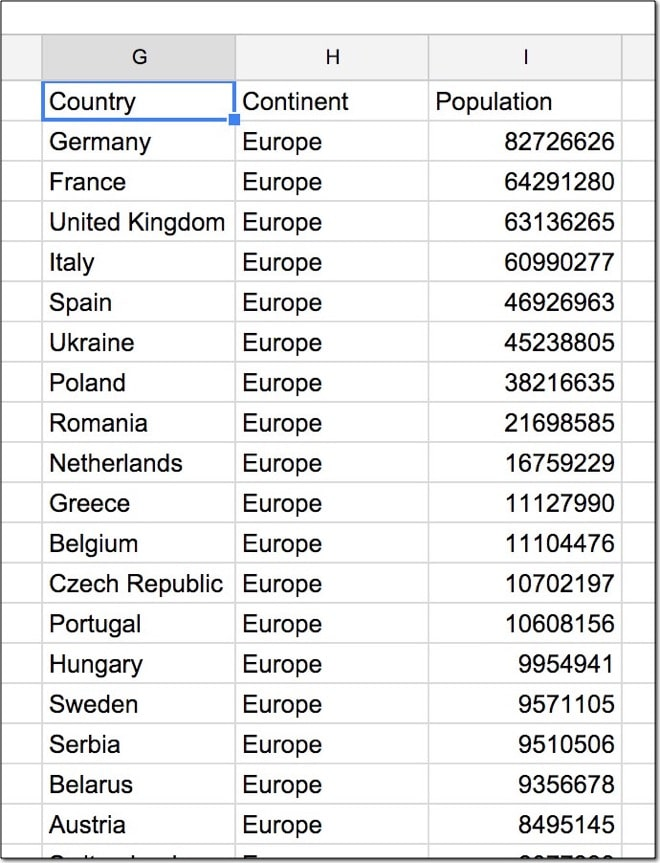Equivalent real world SQL code:

```SELECT country, continent, population FROM countries WHERE continent = 'Europe'```

## ORDER BY clause

The ORDER BY clause sorts our data. We can specify column(s) and direction (ascending or descending). It comes after the SELECT and WHERE clauses.

14. Let’s sort our data by population from smallest to largest. Modify your formula to add the following ORDER BY clause, specifying an ascending direction with ASC:

`=QUERY(countries,"SELECT B, C, D ORDER BY D ASC",1)`

15. The output table: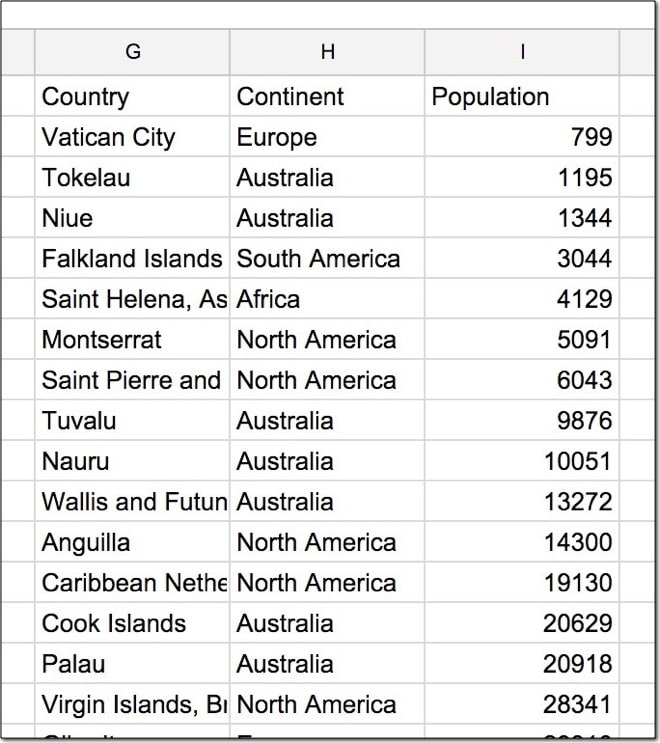Equivalent real world SQL code:

```SELECT country, continent, population FROM countries ORDER BY population ASC```

16. Modify your formula in cell G1 to sort the data by country in descending order, Z – A:

`=QUERY(countries,"SELECT B, C, D ORDER BY B DESC",1)`

17. Output table: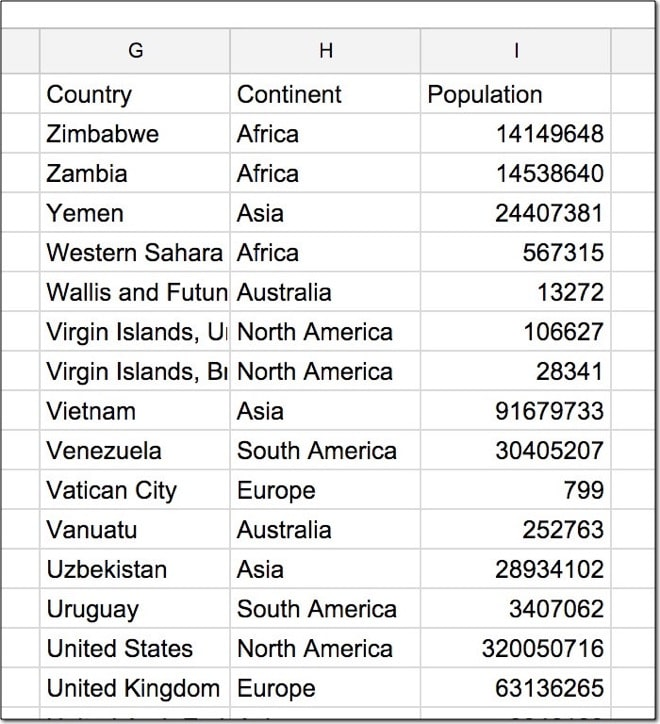Equivalent real world SQL code:

```SELECT country, continent, population FROM countries ORDER BY country DESC```

## LIMIT clause

The LIMIT clause restricts the number of results returned. It comes after the SELECT, WHERE and ORDER BY clauses.

18. Let’s add a LIMIT clause to our formula in G1 and return only 10 results:

`=QUERY(countries,"SELECT B, C, D ORDER BY D ASC LIMIT 10",1)`

19. This now returns only 10 results from our data: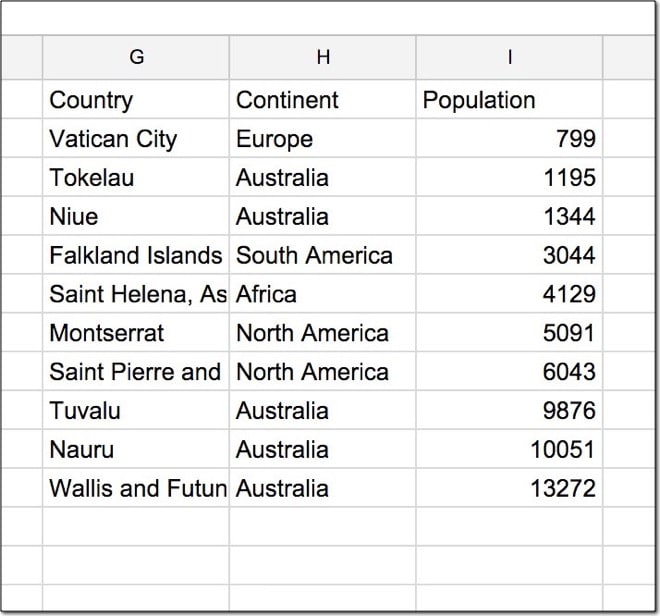Equivalent real world SQL code:

```SELECT country, continent, population FROM countries ORDER BY population ASC LIMIT 10```

## Arithmetic functions

We can perform standard math operations on numeric columns.

So let’s figure out what percentage of the total world population (7.16 billion) each country accounts for.

20. We’re going to divide the population column by the total (7,162,119,434) and multiply by 100 to calculate percentages. So, modify our formula to read:

`=QUERY(countries,"SELECT B, C, (D / 7162119434) * 100",1)`

I’ve divided the values in column D by the total population (inside the parentheses), then multiplied by 100 to get a percentage.

21. The output table this time is: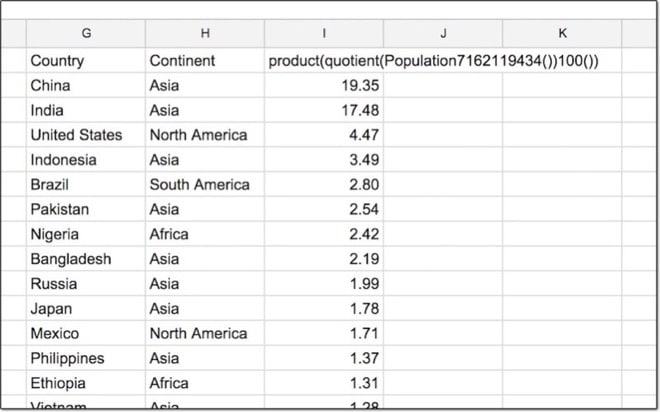Note – I’ve applied formatting to the output column in Google Sheets to only show 2 decimal places.

Equivalent real world SQL code:

```SELECT country, continent, (population / 7162119434) * 100 FROM countries```

## LABEL clause:

22. That heading for the arithmetic column is pretty ugly right? Well, we can rename it using the LABEL clause (however, careful as this is not part of SQL syntax). Try this out:

`=QUERY(countries,"SELECT B, C, (D / 7162119434) * 100 LABEL (D / 7162119434) * 100 'Percentage'",1)`

`=QUERY(countries,"SELECT B, C, (D / 7162119434) * 100 LABEL (D / 7162119434) * 100 'Percentage' ",1)`

Equivalent real world SQL code:

```SELECT country, continent, (population / 7162119434) * 100 AS Percentage FROM countries```

Grab the data and solution file for this tutorial:

## Aggregation functions

We can use other functions in our calculations, for example min, max and average.

23. To calculate the min, max and average populations in your country dataset, use aggregate functions in your query as follows:

`=QUERY(countries,"SELECT max(D), min(D), avg(D)",1)`

24. The output returns three values – the max, min and average populations of the dataset, as follows: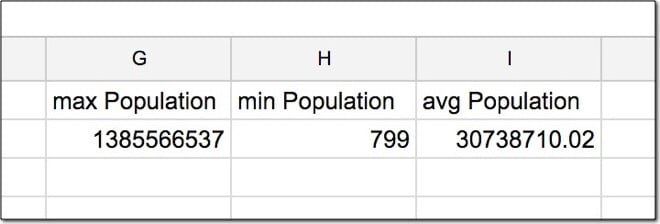Equivalent real world SQL code:

```SELECT max(population), min(population), avg(population) FROM countries```

## GROUP BY clause

Ok, take a deep breath. This is the most challenging concept to understand. However, if you’ve ever used pivot tables in Google Sheets (or Excel) then you should be fine with this.

The GROUP BY clause is used with aggregate functions to summarize data into groups, in the same way a pivot table does.

25. Let’s summarize by continent and count out how many countries per continent. Change your query formula to include a GROUP BY clause and use the COUNT aggregate function to count how many countries, as follows:

`=QUERY(countries,"SELECT C, count(B) GROUP BY C",1)`

Note, every column in the SELECT clause (i.e. before the GROUP BY) must either be aggregated (e.g. counted, min, max) or appear after the GROUP BY clause (e.g. column C in this case).

26. The output for this query is: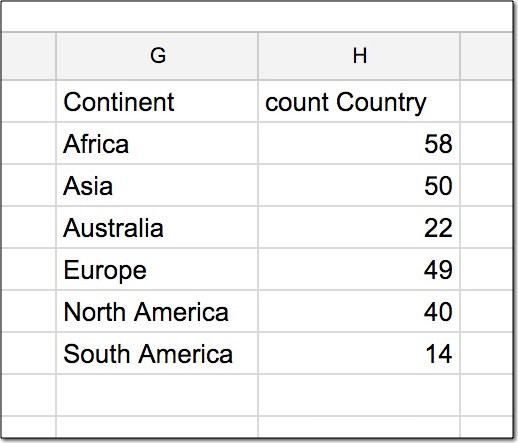Equivalent real world SQL code:

```SELECT continent, count(country) FROM countries GROUP BY continent```

27. Let’s see a more complex example, incorporating many different types of clause. Modify the formula in G1 to read:

`=QUERY(countries,"SELECT C, count(B), min(D), max(D), avg(D) GROUP BY C ORDER BY avg(D) DESC LIMIT 3",1)`

This may be easier to read broken out onto multiple lines:

```=QUERY(countries, "SELECT C, count(B), min(D), max(D), avg(D) GROUP BY C ORDER BY avg(D) DESC LIMIT 3",1)```

This summarizes our data for each continent, sorts by highest to lowest average population and finally limits the results to just the top 3.

The code is very similar to real SQL and it’s looking pretty complex now! Good job!

28. The output of this query is: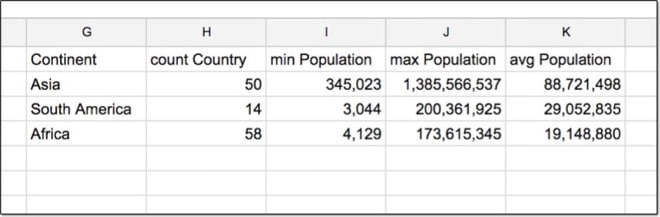Equivalent real world SQL code:

```SELECT continent, count(country), min(population), max(population), avg(population) FROM countries GROUP BY continent ORDER BY avg(population) DESC LIMIT 3```

Well, that’s all for this tutorial folks!

I hope this tutorial allowed you to understand and write some basic SQL code, and see that it needn’t be intimidating.

And I hope you feel inspired to keep learning!

This video is lesson 15 of 30 from my free Google Sheets course: Advanced Formulas 30 Day Challenge.

> How to use dates as filters in your Query formulas